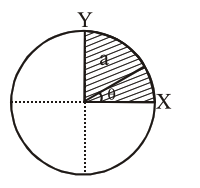# The disc of mass`
Question:

The disc of mass $M$ with uniform surface mass density $\sigma$ is shown in the figure. The centre of mass of the quarter disc (the shaded area) is at the position $\frac{\mathrm{x}}{3} \frac{\mathrm{a}}{\pi}, \frac{\mathrm{x}}{3} \frac{\mathrm{a}}{\pi}$ where $\mathrm{x}$ is___________

(Round off to the Nearest Integer)

[a is an area as shown in the figure]Solution:

C.O.M of quarter disc is at $\frac{4 a}{3 \pi}, \frac{4 a}{3 \pi}$

$=4$